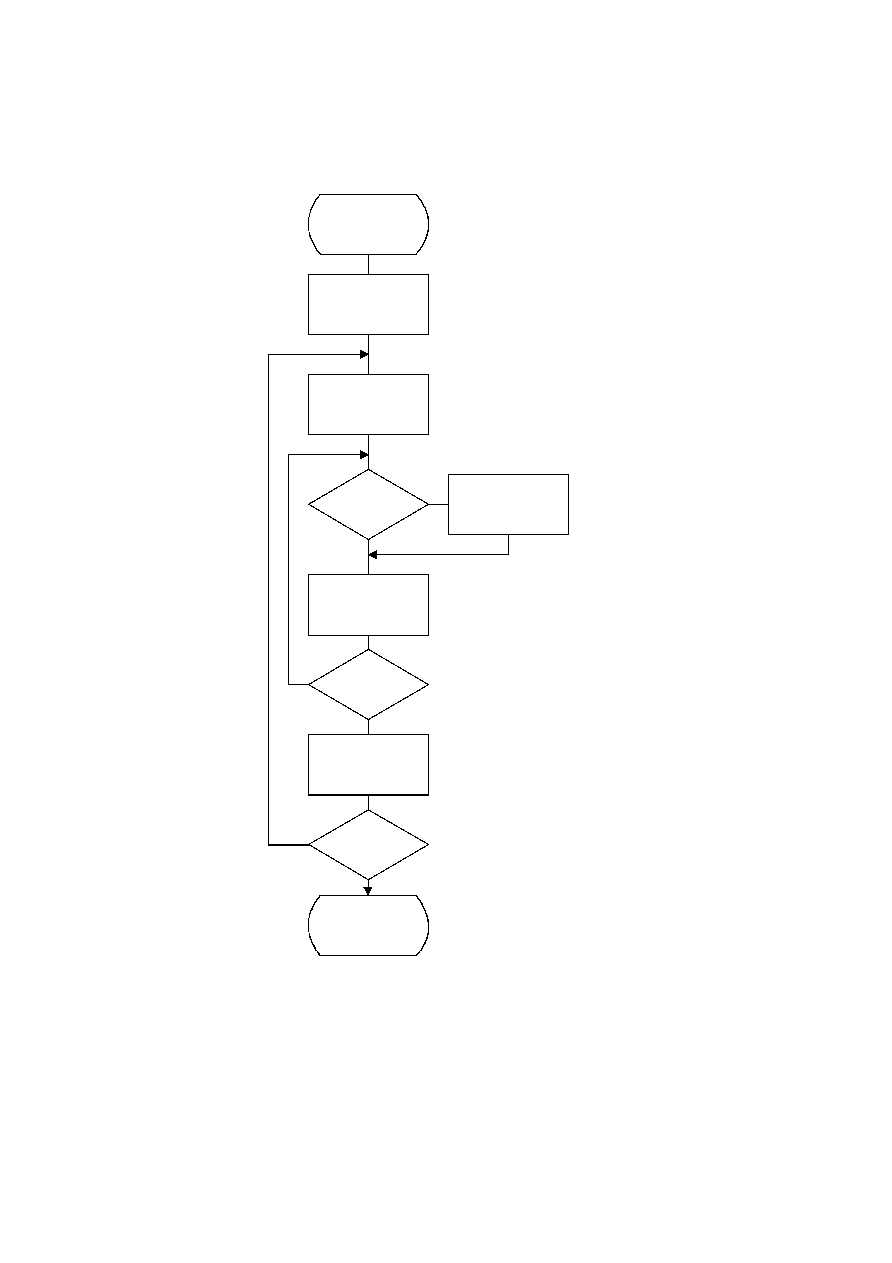ISO/IEC 10918-1 : 1993(E)
The input values are sorted according to code size as shown in Figure K.4. HUFFVAL is the list containing the input
values associated with each code word, in order of increasing code length.
At this point, the list of code lengths (BITS) and the list of values (HUFFVAL) can be used to generate the code tables.
These procedures are described in Annex C.
TISO1760-93/d114
Sort_input
J = 0
J = J + 1
I = I + 1
Done
Yes
No
Yes
No
No
Yes
HUFFVAL(K) = J
K = K + 1
I = 1
K = 0
CODESIZE(J) = I
?
J > 255
?
I > 32
?
Figure K.4 ­ Sorting of input values according to code size
Figure K.4 [D114] = 20.5 cm = 801 %
K.3
Typical Huffman tables for 8-bit precision luminance and chrominance
Huffman table-specification syntax is specified in B.2.4.2.
148
CCITT Rec. T.81 (1992 E)